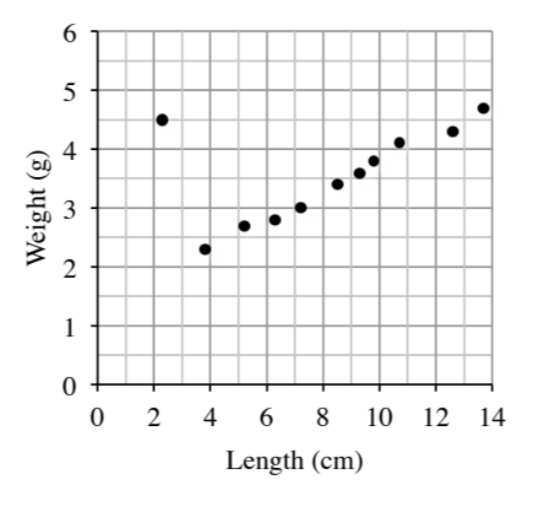### Home > GB8I > Chapter 4 Unit 5 > Lesson INT1: 4.1.1 > Problem4-4

4-4.

Sam collected data by measuring the pencils of her classmates. She recorded the length of the painted part of each pencil and its weight. Her data is shown on the graph at right.

1. Describe the association between weight and length of the painted part of the pencil. Remember to describe the form, direction, strength, and outliers.

What shape do the points on the graph form? This is the form. Be sure to describe the direction, strength, and outliers.

There is a strong positive linear association with an apparent outlier at $2.3$ cm.

2. Make a conjecture (a guess) about why Sam’s data had an outlier.

How could you change the outlying point to make it correspond with the other points on the graph.

3. Sam created a line of best fit: $w=1.4+0.25l$ where $w$ is the weight of the pencil in grams and $l$ is the length of the paint part on the pencil in centimeters. What does the slope represent in this context?• Which number in the given equation represents the slope? What would the units of the slope be in this situation?

An increase of $1$ cm in length is expected to increase the weight by $0.25$ g.

1. Sam’s teacher has a pencil where the painted part is $11.5$ long.  Predict the weight of the teacher’s pencil.

Let $l = 11.5$ in the equation given in part (c).

2. Interpret the meaning of the $y$-intercept in context.

• The y-intercept in this situation is predicted to be $(0, 1.4)$. What are the units for $x$ and $y$?

When the pencil is so short that there is no paint left, we predict that it will weigh $1.4$ g.